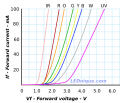# Calculating Voltage

#### Papabravo

Joined Feb 24, 2006
18,432
We don't generally calculate the voltage across an LED. We look the manufacturer's datasheet and based on the current through the LED we estimate the forward voltage drop. The forward voltage drop of an LED that is on won't change much as the current varies over a wide range.

#### Alec_t

Joined Sep 17, 2013
12,818
According to the schematic the series combination of the LED and the 1k resistor has only 903mV across it. The voltage across the LED (not in the LED) will be 903mV less the voltage drop across that resistor. Now you just need to find the current tthrough that resistor and use Ohm's Law.

#### Polipker

Joined May 11, 2022
9
According to the schematic the series combination of the LED and the 1k resistor has only 903mV across it. The voltage across the LED (not in the LED) will be 903mV less the voltage drop across that resistor. Now you just need to find the current tthrough that resistor and use Ohm's Law.
Thanks you mate!

#### Audioguru again

Joined Oct 21, 2019
4,693
An LED will not conduct with such a low voltage as 903mV.
The transistor driving that LED shows a current of 4.53mA then maybe the voltmeter has a very low resistance and is using most of the transistor current.

#### MrChips

Joined Oct 2, 2009
26,133
An LED is a PN junction semiconductor device. This has an exponential I-V relationship.
The slope of the I-V curves represent the reciprocal of the resistance, i.e. 1/R.

Hence you can see that when Vf is below 1V the slope is almost zero, thus the resistance is very high.
With 903mV bias voltage on the LED and 1kΩ series resistor, almost all of the 903mV will be across the LED.